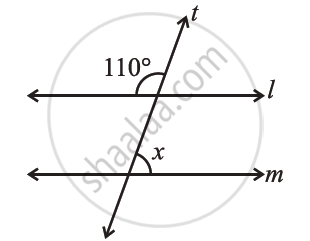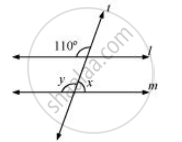Share

# Find the Value of X in the Following Figures If L Parallel M. - CBSE Class 7 - Mathematics

ConceptPairs of Lines - Intersecting Lines

#### Question

Find the value of x in the following figures if l || m.#### Solutiony = 110° (Corresponding angles)

x + ∠y = 180° (Linear pair)

y = 180° − 110°

= 70°

Is there an error in this question or solution?

#### APPEARS IN

NCERT Solution for Mathematics for Class 7 (2018 to Current)
Chapter 5: Lines and Angles
Ex. 5.20 | Q: 4.1 | Page no. 111
Solution Find the Value of X in the Following Figures If L Parallel M. Concept: Pairs of Lines - Intersecting Lines.
S